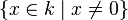# General linear group over a field as an algebraic group

## Definition

Suppose$k$ is a field and$n$ is a natural number. The general linear group$GL(n,k)$ is defined as the group of all invertible$n \times n$ matrices over$k$. This has the natural structure of an algebraic group as described below:

• The algebraic variety structures arises as follows: it is a Zariski-open subset of$k^{n^2}$, defined by the non-vanishing of the determinant polynomial.
• The group structure is by matrix multiplication. Both the matrix multiplication and the inverse map are given globally by rational functions in the coordinates, and hence are morphisms of algebraic varieties.

## Particular cases

### General linear group of degree one as an algebraic group

For$n = 1$, the general linear group$GL(1,k)$ is the subset$k^\ast$ of the field$k$. Explicitly:

Item Description Comment
Definition as Zariski-open subset of$k^{1^2} = k$$\{ x \in k \mid x \ne 0 \}$
Explicit formula for group multiplication$(x,y) \mapsto xy$ Group multiplication is field multiplication.
Explicit formula for inverse map in group$x \mapsto 1/x$ Group inverse is field inverse.

### General linear group of degree two as an algebraic group

Here, we write a matrix$\begin{pmatrix} x_{11} & x_{12} \\ x_{21} & x_{22} \\\end{pmatrix}$ as a bunch of four coordinates$(x_{11}, x_{12}, x_{21}, x_{22})$. Then:

Item Description Comment
Definition as Zariski-open subset of$k^{2^2} = k^4$$\{ (x_{11},x_{12},x_{21},x_{22}) \in k^4 \mid x_{11}x_{22} - x_{21}x_{12} \ne 0 \}$ Nonvanishing determinant condition
Explicit formula for group multiplication$((x_{11},x_{12},x_{21},x_{22}),(y_{11},y_{12},y_{21},y_{22})) \mapsto (x_{11}y_{11} + x_{12}y_{21}, x_{11}y_{12} + x_{12}y_{22}, x_{21}y_{11} + x_{22}y_{12}, x_{21}y_{12} + x_{22}y_{22})$ Group multiplication is matrix multiplication.
Explicit formula for inverse map in group$(x_{11},x_{12},x_{21},x_{22}) \mapsto (x_{22}/(x_{11}x_{22} - x_{21}x_{12}), -x_{12}/(x_{11}x_{22} - x_{21}x_{12}), -x_{21}/(x_{11}x_{22} - x_{21}x_{12}),x_{11}/(x_{11}x_{22} - x_{21}x_{12}))$ Group inverse is matrix inverse. Note that denominators do not vanish, and this is precisely because the determinant is nonvanishing on this open subset.